Conjugate

Chapter 4 Class 11 Complex Numbers
Concept wise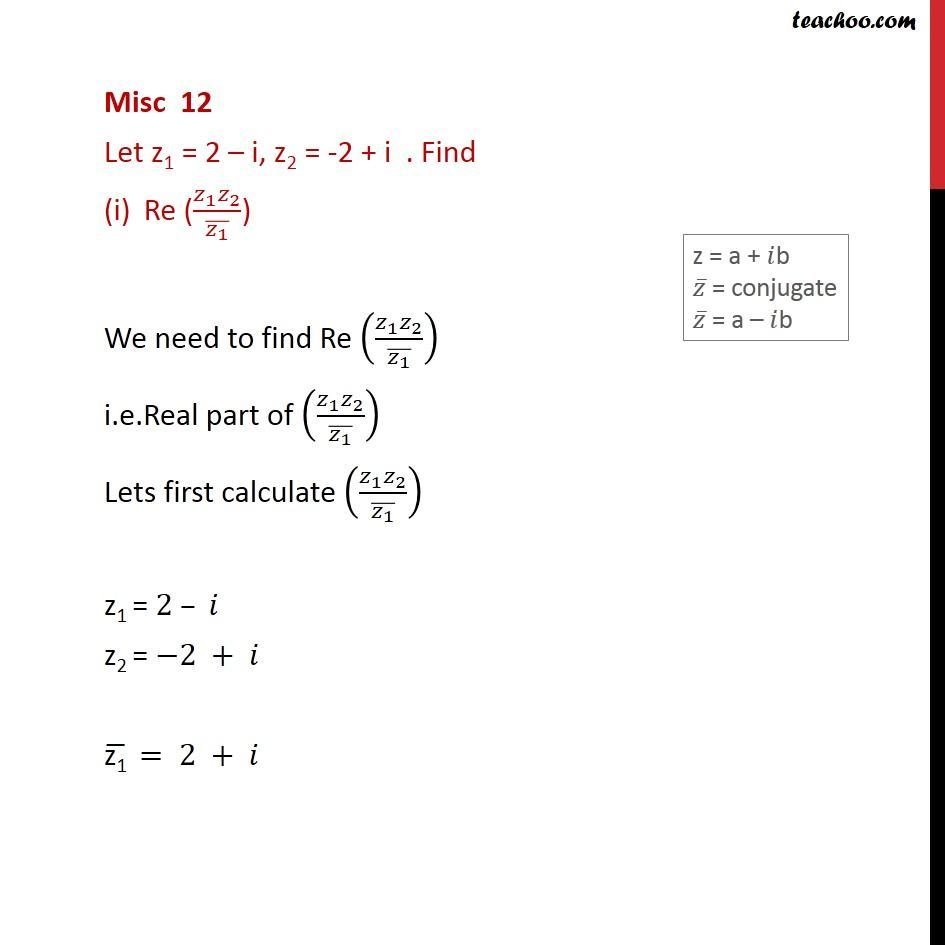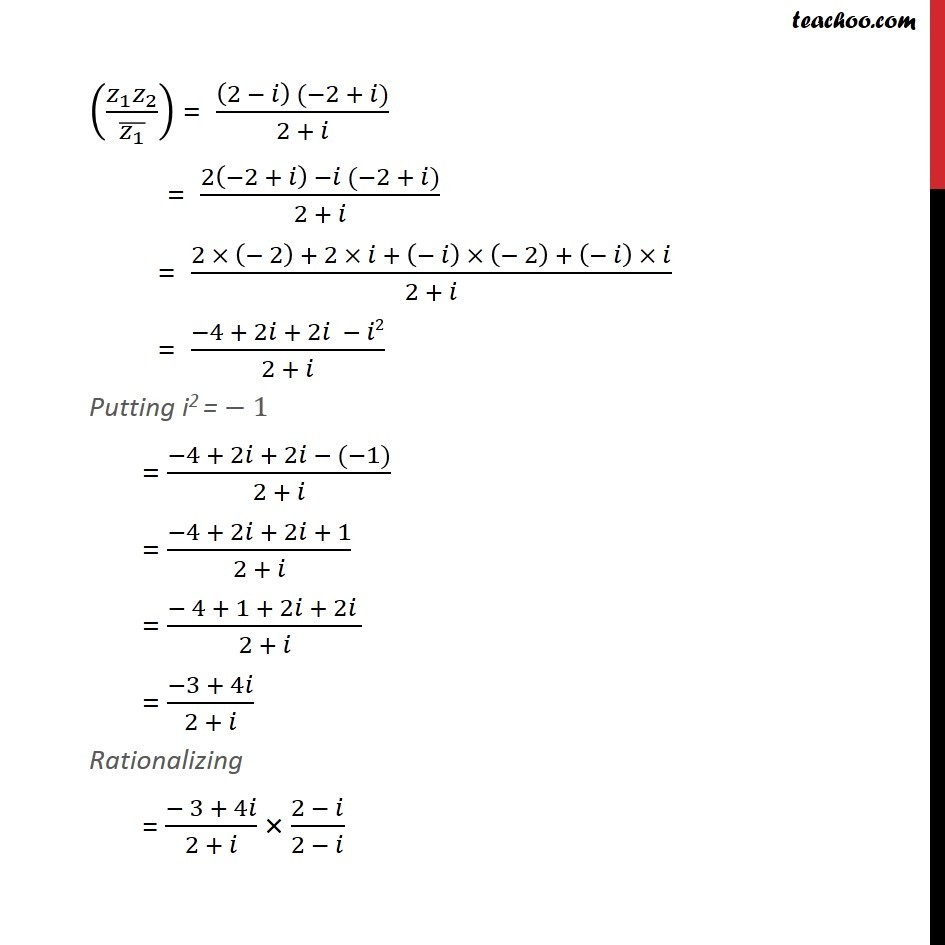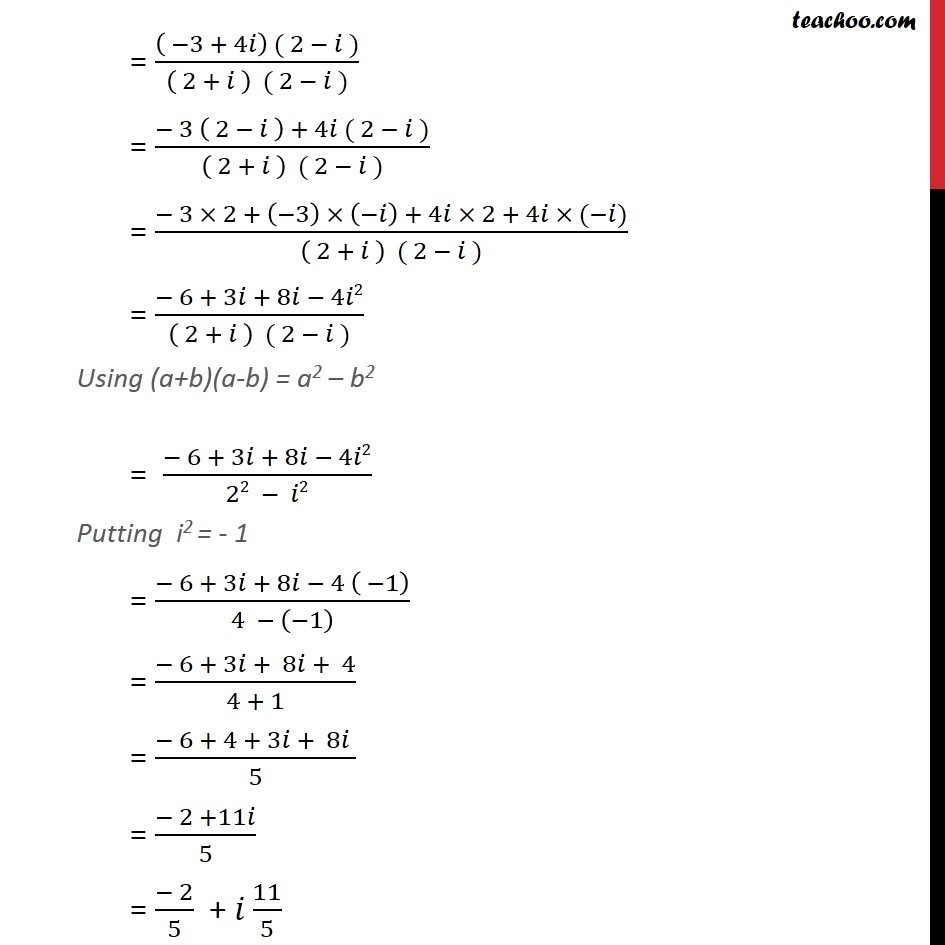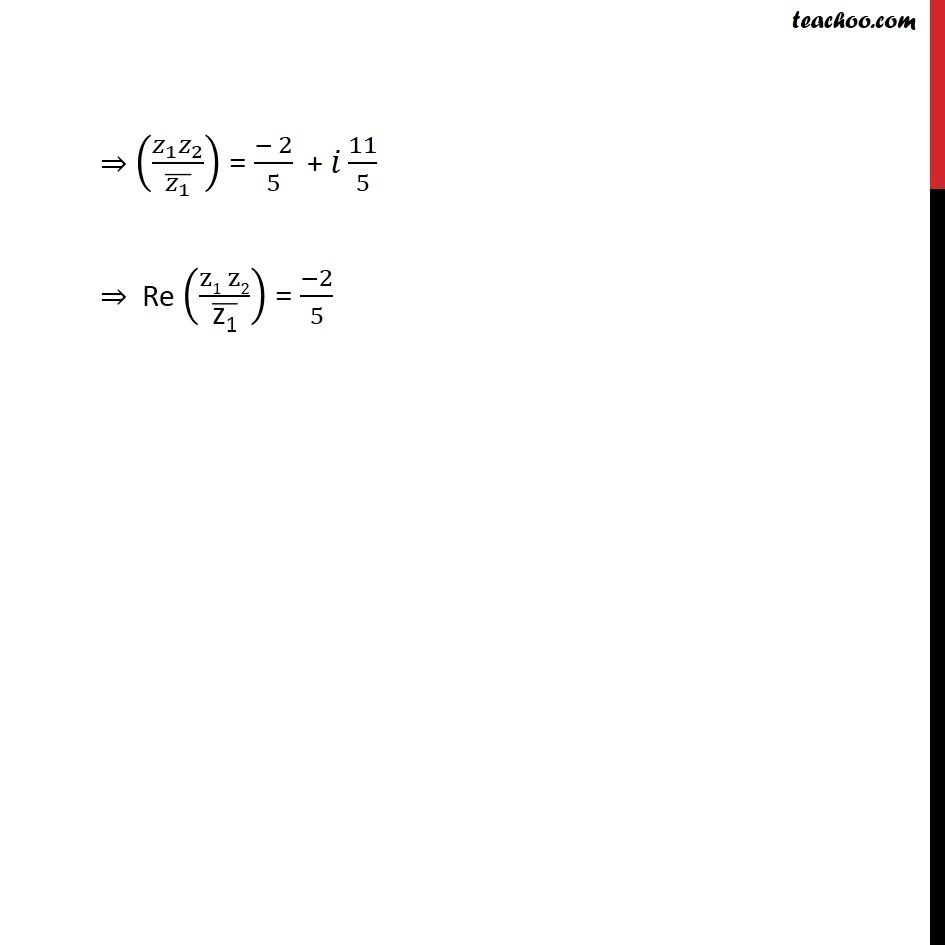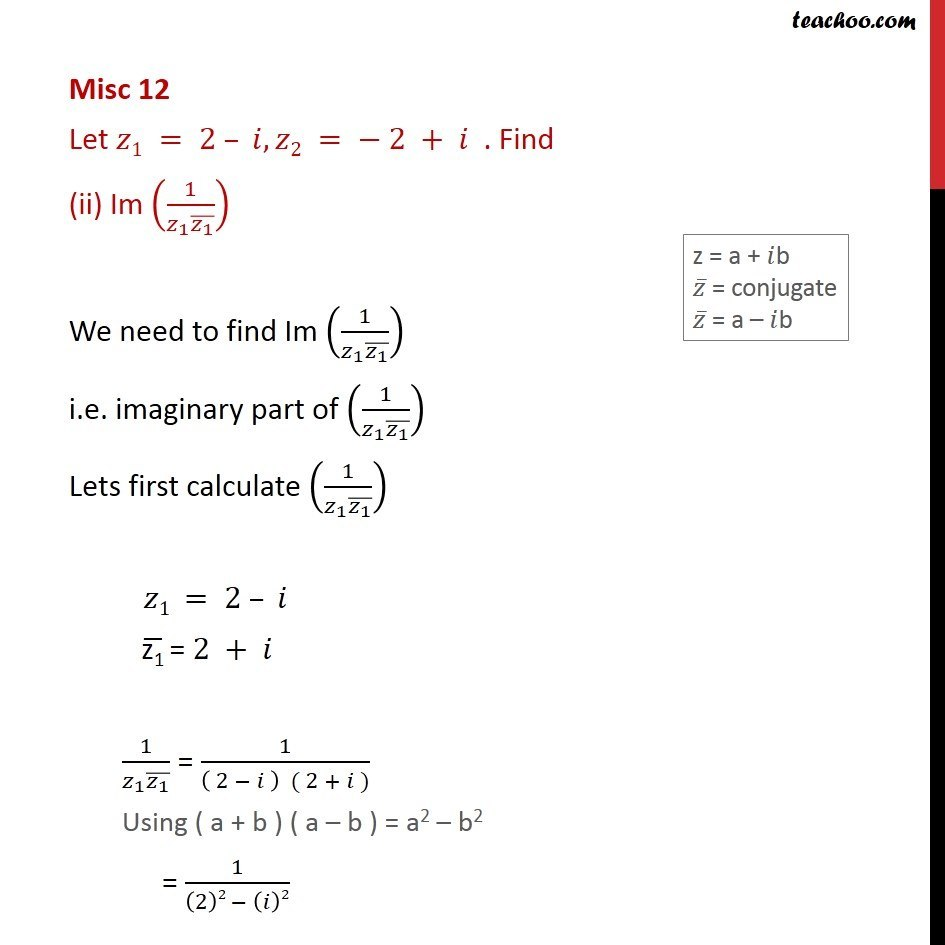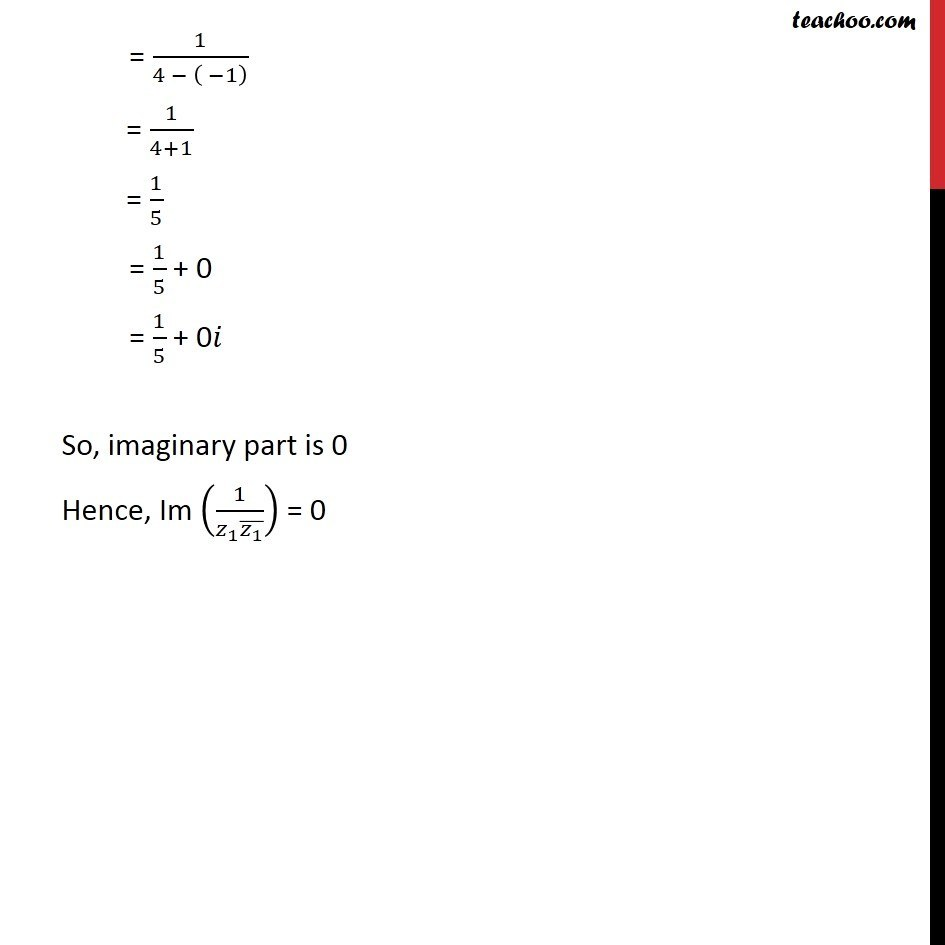Learn in your speed, with individual attention - Teachoo Maths 1-on-1 Class

### Transcript

Misc 12 Let z1 = 2 i, z2 = -2 + i . Find Re (( _1 _2)/( _1 ) ) We need to find Re (( _1 _2)/( _1 ) ) i.e.Real part of (( _1 _2)/( _1 ) ) Lets first calculate (( _1 _2)/( _1 ) ) z1 = 2 z2 = 2 + ("z1" ) = 2 + (( _1 _2)/( _1 ) ) = ((2 ) ( 2 + ))/(2 + ) = (2( 2 + ) ( 2 + ))/(2 + ) = (2 ( 2) + 2 + ( ) ( 2) + ( ) )/(2 + ) = ( 4 + 2 + 2 2)/(2 + ) Putting i2 = 1 = ( 4 + 2 + 2 ( 1))/(2 + ) = ( 4 + 2 + 2 + 1)/(2 + ) = ( 4 + 1 + 2 + 2 )/(2 + ) = ( 3 + 4 )/(2 + ) Rationalizing = ( 3 + 4 )/(2 + ) (2 )/(2 ) = (( 3 + 4 ) ( 2 ))/(( 2 + ) ( 2 )) = ( 3 ( 2 ) + 4 ( 2 ))/(( 2 + ) ( 2 )) = ( 3 2 + ( 3) ( ) + 4 2 + 4 ( ))/(( 2 + ) ( 2 )) = ( 6 + 3 + 8 4 2)/(( 2 + ) ( 2 )) Using (a+b)(a-b) = a2 b2 = ( 6 + 3 + 8 4 2)/(22 2) Putting i2 = - 1 = ( 6 + 3 + 8 4 ( 1))/(4 ( 1) ) = ( 6 + 3 + 8 + 4)/(4 + 1) = ( 6 + 4 + 3 + 8 )/5 = ( 2 +11 )/5 = ( 2)/5 + 11/5 (( _1 _2)/( _1 ) ) = ( 2)/5 + 11/5 Re ((z1 z2)/("z1" ) ) = ( 2)/5 Misc 12 Let 1 = 2 , 2 = 2 + . Find (ii) Im (1/( _1 ( _1 ) )) We need to find Im (1/( _1 ( _1 ) )) i.e. imaginary part of (1/( _1 ( _1 ) )) Lets first calculate (1/( _1 ( _1 ) )) 1 = 2 ("z1" ) = 2 + 1/( _1 ( _1 ) ) = 1/(( 2 ) ( 2 + )) Using ( a + b ) ( a b ) = a2 b2 = 1/((2)2 ( )2) = 1/(4 ( 1) ) = 1/(4+1) = 1/5 = 1/5 + 0 = 1/5 + 0 So, imaginary part is 0 Hence, Im (1/( _1 ( _1 ) )) = 0